IF Function in Microsoft Excel 2013

In an If…Then…Else statement, one or more conditions are checked.  If the condition is found to be True, the statements following the if statement are executed. If the condition is found to be False, each Else If statement (if any) is evaluated in order. When a True else-if condition is found, the statements immediately following the associated Else-If are executed. If there are no Else-If statements, the statements following Else are executed.

The IF condition is acommonly used function in Excel that you are likely to come across.It becomes important that you know how to write these statements.

You may also need to write a Nested IF statement which are complicated to look at, but are simple the moment you understand the concept.

A Nested IF statement will look like this:

=IF(“if this condition stated here is true”, then enter “this value, else if(“if this condition stated here is true”, then enter “this value, else enter “this value”))

Now lets understand the If function.

IF: Checks whether a condition is met, and returns one value if TRUE, and another value if FALSE

Syntax =IF(logical_test,value_if_true,value_if_false)

Parameters:

Logical test is the condition or a value that you want to test.

value_if_true is optional. It is the value that is returned if the condition is TRUE.

value_if_false is optional. It is the value that is returned if the condition is FALSE.

For example – We have marks in column A and we need to get the Grade in column B.  The various grades are given below -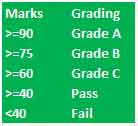• If the marks are greater than or equal to 90, the function returns Grade A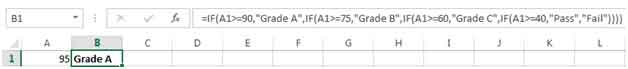• If the marksare greater than or equal to 75, the function returns Grade B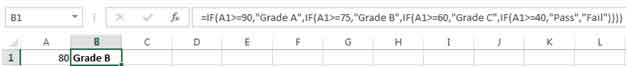• If the marksare greater than or equal to 60, the function returns Grade C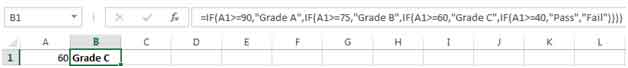• If the marks are greater than or equal to 40, the function returns Pass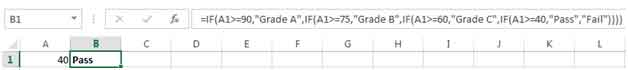• If the marks are less than 40, the function returns Fail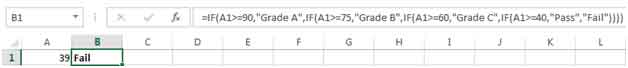Lets take another Example of Nested if to understand more:

• Column A contains Dates
• Today’s date is 24-Jun-2014
• Column B will contain theresult as shown in the below screenshot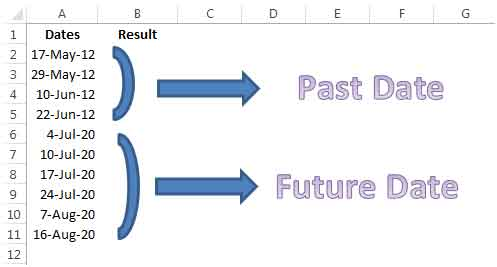• The formula in cell B2=IF (A2>TODAY (),”Future Date”,IF(A2<TODAY(),”Past Date”,”Today”))
• The If condition will check if the Date in cell A2 is greater than today’s date
• If the condition is found to be true, then it will proceed further &it will return “Future Date” in column B.
• If the condition is found to be false, thenit will jump to the next if condition & check if that is true or false & return the output.  So in the 2nd if condition, it will check if the Date is less than today’s date.  If it is true, it will return “Past Date”, else it will return “Today” in column B.
• You will receive the results as below –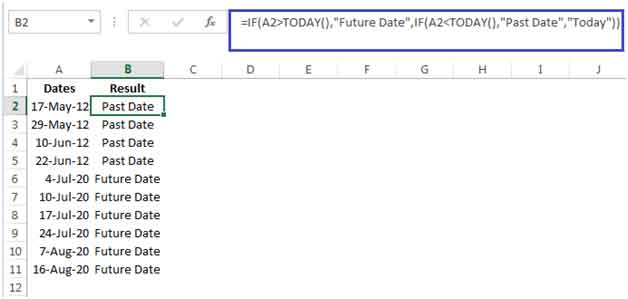## Users are saying about us...

1.Are these available to be saved and printed out?

#### Categories

Terms and Conditions of use

The applications/code on this site are distributed as is and without warranties or liability. In no event shall the owner of the copyrights, or the authors of the applications/code be liable for any loss of profit, any problems or any damage resulting from the use or evaluation of the applications/code.